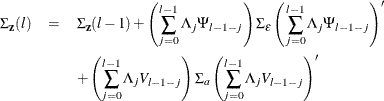The VARMAX Procedure

Forecasting

Subsections:

The optimal (minimum MSE) l-step-ahead forecast of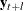is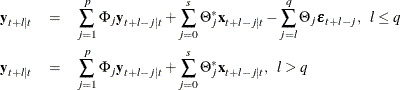with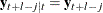and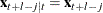for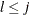. For the forecasts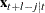, see the section State-Space Representation.

Covariance Matrices of Prediction Errors without Exogenous (Independent) Variables

Under the stationarity assumption, the optimal (minimum MSE) l-step-ahead forecast ofhas an infinite moving-average form,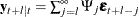. The prediction error of the optimal l-step-ahead forecast is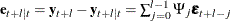, with zero mean and covariance matrix,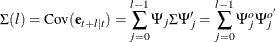where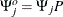with a lower triangular matrix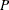such that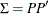. Under the assumption of normality of the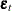, the l-step-ahead prediction error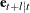is also normally distributed as multivariate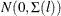. Hence, it follows that the diagonal elements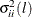of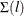can be used, together with the point forecasts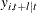, to construct l-step-ahead prediction intervals of the future values of the component series,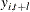.

The following statements use the COVPE option to compute the covariance matrices of the prediction errors for a VAR(1) model. The parts of the VARMAX procedure output are shown in Figure 35.36 and Figure 35.37.

proc varmax data=simul1;
model y1 y2 / p=1 noint lagmax=5
printform=both
print=(decompose(5) impulse=(all) covpe(5));
run;


Figure 35.36 is the output in a matrix format associated with the COVPE option for the prediction error covariance matrices.

Figure 35.36: Covariances of Prediction Errors (COVPE Option)

The VARMAX Procedure

Prediction Error Covariances
1 y1 1.28875 0.39751
y2 0.39751 1.41839
2 y1 2.92119 1.00189
y2 1.00189 2.18051
3 y1 4.59984 1.98771
y2 1.98771 3.03498
4 y1 5.91299 3.04856
y2 3.04856 4.07738
5 y1 6.69463 3.85346
y2 3.85346 5.07010

Figure 35.37 is the output in a univariate format associated with the COVPE option for the prediction error covariances. This printing format more easily explains the prediction error covariances of each variable.

Figure 35.37: Covariances of Prediction Errors

Prediction Error Covariances by Variable
y1 1 1.28875 0.39751
2 2.92119 1.00189
3 4.59984 1.98771
4 5.91299 3.04856
5 6.69463 3.85346
y2 1 0.39751 1.41839
2 1.00189 2.18051
3 1.98771 3.03498
4 3.04856 4.07738
5 3.85346 5.07010

Covariance Matrices of Prediction Errors in the Presence of Exogenous (Independent) Variables

Exogenous variables can be both stochastic and nonstochastic (deterministic) variables. Considering the forecasts in the VARMAX(p,q,s) model, there are two cases.

When exogenous (independent) variables are stochastic (future values not specified):

As defined in the section State-Space Representation,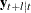has the representation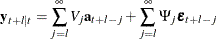and hence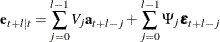Therefore, the covariance matrix of the l-step-ahead prediction error is given as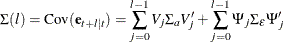where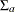is the covariance of the white noise series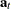, andis the white noise series for the VARMA(p,q) model of exogenous (independent) variables, which is assumed not to be correlated withor its lags.

When future exogenous (independent) variables are specified:

The optimal forecastof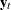conditioned on the past information and also on known future values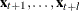can be represented as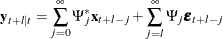and the forecast error is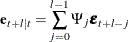Thus, the covariance matrix of the l-step-ahead prediction error is given as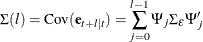Decomposition of Prediction Error Covariances

In the relation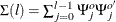, the diagonal elements can be interpreted as providing a decomposition of the l-step-ahead prediction error covariance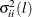for each component series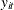into contributions from the components of the standardized innovations.

If you denote the (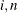)th element of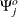by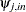, the MSE of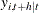is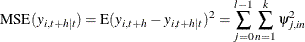Note that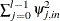is interpreted as the contribution of innovations in variable n to the prediction error covariance of the l-step-ahead forecast of variable i.

The proportion,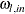, of the l-step-ahead forecast error covariance of variable i accounting for the innovations in variable n is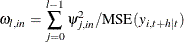The following statements use the DECOMPOSE option to compute the decomposition of prediction error covariances and their proportions for a VAR(1) model:

proc varmax data=simul1;
model y1 y2 / p=1 noint print=(decompose(15))
printform=univariate;
run;


The proportions of decomposition of prediction error covariances of two variables are given in Figure 35.38. The output explains that about 91.356% of the one-step-ahead prediction error covariances of the variable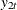is accounted for by its own innovations and about 8.644% is accounted for by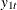innovations.

Figure 35.38: Decomposition of Prediction Error Covariances (DECOMPOSE Option)

Proportions of Prediction Error Covariances
by Variable
y1 1 1.00000 0.00000
2 0.88436 0.11564
3 0.75132 0.24868
4 0.64897 0.35103
5 0.58460 0.41540
y2 1 0.08644 0.91356
2 0.31767 0.68233
3 0.50247 0.49753
4 0.55607 0.44393
5 0.53549 0.46451

Forecasting of the Centered Series

If the CENTER option is specified, the sample mean vector is added to the forecast.

Forecasting of the Differenced Series

If dependent (endogenous) variables are differenced, the final forecasts and their prediction error covariances are produced by integrating those of the differenced series. However, if the PRIOR option is specified, the forecasts and their prediction error variances of the differenced series are produced.

Let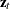be the original series with some appended zero values that correspond to the unobserved past observations. Let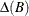be the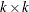matrix polynomial in the backshift operator that corresponds to the differencing specified by the MODEL statement. The off-diagonal elements of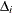are zero, and the diagonal elements can be different. Then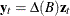.

This gives the relationship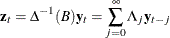where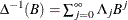and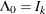.

The l-step-ahead prediction of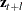is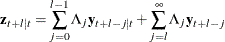The l-step-ahead prediction error ofis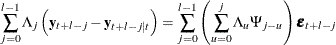Letting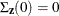, the covariance matrix of the l-step-ahead prediction error of,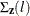, is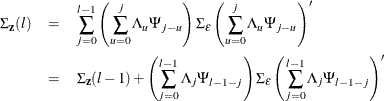If there are stochastic exogenous (independent) variables, the covariance matrix of the l-step-ahead prediction error of,, is